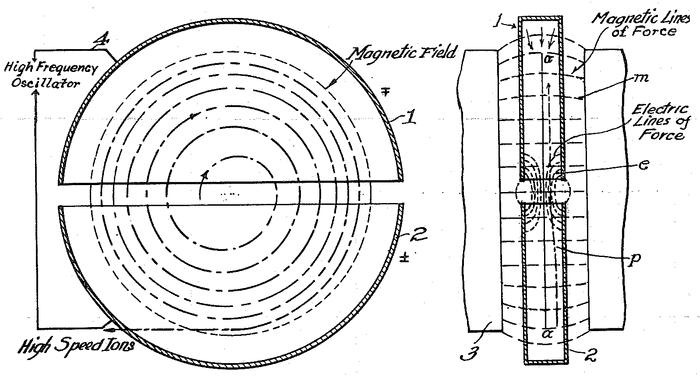# Electric Field In A Cyclotron

• Aatif Moin

#### Aatif Moin

The E-field originating from the opposite surfaces (above and below) cancel out one another but what about the fields originating from the circular sides? Why is the electric field inside the cyclotron zero then?

•Symmetry777
The sides are far away from the region where particles are accelerated - far enough to be neglected unless you actually build one.
The relevant ratio here is the distance to the edge divided by the distance between the plates - if this ratio is much larger than 1, you can neglect the remaining field between the electrodes.

But if the E-field is negligible in the space between the Dees, what accelerates the charges? Also, the E-field "inside" is required to be zero / negligible, not in the space.

Last edited:
Timing is everything in RF particle accelerators.

Acceleration takes place in the space between the Dees. The electric field there is alternating with RF, so the accelerator cannot produce a continuous DC current. Rather, small bunches of particles get accelerated if they are times such that each time the cross the gap the voltage has the correct sign. This can only happen if the time it takes to complete the half circle inside the Dee is the same as the half period of the RF accelerating voltage, i.e. the RF frequency has to match the mass of the particle and the strength of the magnetic field (these two give the radius of curvature of the particle's track)

@M Quack - So, how does the E-field inside the Dees cancel?

The height of the Dees is much smaller than their diameter, and the gap is smaller than the height. If you just statically charged the dees, one positive and one negative, what would the field lines look like?

the field lines would originate from one dee and end-up at another.....they still would'nt cancel out. Moreover, if they are kept statically charged, the B- field would make the particles collide with the floor.

If the frequency is low enough, then you can approximate the field in and in between the Dees as electrostatic fields that vary slowly as the applied voltage is changed.

You were asking about the electric field inside the Dees. What would the field lines look like there?

Ok, the field lines inside the dees would, as I said, look as if an arrow is shot from the +vely charged dee into the -vely charged dee

@Aatif Moin: I thought you were asking about the space inside the dees (where no acceleration happens), not between them (= in the acceleration gap).
Inside, the particles are surrounded by two plates of the same potential, the electric field is nearly zero.
Between them (in the acceleration gap), they go from a high potential energy to a low potential energy - they get accelerated along the field lines they follow.

If I'm getting u right, u mean there are "no" edge walls, meaning that the dees are actually made up of 2 plates above and below and not "around" them.

It does not matter much, at least for the field distribution. There are certainly no walls in the acceleration gap, of course.

man, leave the acceleration gap. And, i think it does matter because if the edges are metallic, they'll produce a field radially which would account for an ineffective shielding - effect. By the way if there are no edge walls, how do the charges get accelerated in the acceleration - gap...i mean how do the charges find their way?

i mean how do the charges find their way?
Where would you expect them to go?
They mainly go in circles due to the magnetic field.

They go in circles, in a plane parallel to the plates of the dees if ,and only if, the field inside the dees is zero. If it is not , they will collide with the lower / upper plate....the field inside has to be purely magnetic.

if ,and only if, the field inside the dees is zero.
It is, because both plates are at the same potential.

It is, because both plates are at the same potential.
what do u mean?

Which part is unclear?

In short , can you describe the fields in a cyclotron, especially the E- fields...how are they created and cancelled....if you cannot just tell me "how" are they cancelled?

It is all in the thread now.

You have three regions:
Inside dee 1, the particle is surrounded by conducting plates of the same potential, so the electric field at the place of the particles is negligible (the potential is the same everywhere around them).
Inside dee 2, the particle is surrounded by conducting plates of the same potential, so the electric field at the place of the particles is negligible (the potential is the same everywhere around them).
Between the dees (at the time the particles are there), there is an electric field from one dee to the other as the plates are at a different potential.

@mfb Well, sir, I'm sorry if I'm asking too much but can u get me a picture of the e-field inside a cyclotron......I'll be grateful.

Google has tons of them. Just two dees with an electric field from one to the other.yeah, I got it now. Those are the "fringing fields" which make-up the e-fields ... not the fields entire body

•Ajay Singh